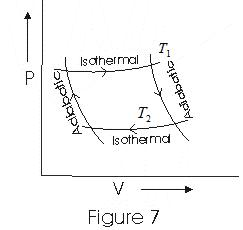# Thermodynamics

## 14. Carnot's Heat Engine

• According to second law of thermodynamics, no heat engine can have 100% efficiency
• Carnot�s heat engine is an idealized heat engine that has maximum possible efficiency consistent with the second law.
• Cycle through which working substance passed in Carnot�s engine is known as Carnot�s Cycle.
• Carnot's engine works between two temperatures
T1 - temperature of hot reservoir
T2 - temperature of cold reservoir
• In a Complete Carnot's Cycle system is taken from temperature T1 to T2 and then back from
temerature T2 to T1.
• We have taken ideal gas as the working substance of cornot engine.
• Fig below is an indicator digram for Cornot Cycle of an ideal gas(i) In step b→c iso thermal esepansion of gas taken place and thermodynamic variables of gas changes from (P1, V1,T1) to (P2,V2,T1)
• If Q1 is the amount of heat absorbed by working substance from the source and W1 the work done by the gas then from eqn (13)
Q1 = W1 = nRT1 ln (V2/V1)     (19)
as process is iso thermal.
(ii) Step c→d is an adiabatic expension of gas from (P2, V2, T1) to (P3,V3,T2). Work done by gas in adiabatic esepansion is given by eqn (16)
W2 = nR (T1-T2)/(γ-1)               (20)
(iii)Step d→a is iso-thermal compression of gas from (P3,V3,T2) to (P4,V4,T2). Heat Q2 would be released by the gas to the at temperature T2
• Work done on the gas by the environment is
W3 = Q2
= nRT2ln(V3/ V4)               (21)
(iv)Step a→b is adiabatic compression of gas from (P4, V4, T2) to (P1, V1, T1)
• Work done on the gas is
W4 =nR (T1-T2)/(γ-1)                    (22)
• Now total work done in one complete cycle is
W = W1 + W2 - W3 - W4
= nRT1ln(V2/V1)-nRT2ln(V3/V4)          (23)
as W2 = W4

• Efficency of carnot engine
η=W/Q1 = 1-(Q2/Q1)
= 1-(T2/T1)ln(V3/V4)/ln(V2/V1)          (24)
or     η= 1-[T2ln(V3/V4)/T1ln(V2/V1)]          (25)
Since points b and c lie on same iso thermal
⇒ P1V1=P2V2                    (26)
also points c and d lie on same adiabatic
⇒     P2(V2)γ=P3(V3)γ                    (27)
also points d and a lie on same iso thermal and points a and b on sum adiabatic thus,
P3V3=P4V4                    (28)
P4(V4)γ=P2(V1)γ                         (29)
multiplying all the above four eqns me get
V3/4 = V2/V1                    (30)
Putting this in equation (25) we get
η= 1-(T2/T1)                         (31)
From above eqn we can draw following conclusions that efficency of Carnot engine is
(i) independent of the nature of working substance
(ii) depend on temperature of source and sink

## 15. Carnot Theorem

• Carnot Engine is a reversible engine.
• Carnot�s theorem consists of two parts
(i) no engine working between two given temperatures can be more efficent than a reversible Carnot engine working between same source and sink.
(ii) all reversible engines working between same source and sink (same limits or temperature) have the same efficiency irrespective the working substance.